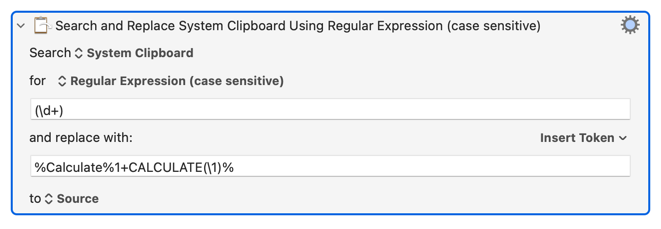# Can I Add 1 to the Number in the Clipboard and Paste It?

I am having trouble using search and replace. I want to copy text that has numbers and strings attached to it, and when I paste it, I want the numbers to be incremented by 1. For example, if I copy 1W, then 2W to paste, then 3W to paste again, and so on.The replacement is:

%Calculate%1+CALCULATE(\1)%

So search for the number, and then use the Calculate token to calculate 1+ the matched value. The matched value is itself only available as a token, so use the CALCULATE function to get the resulting text as a numeric result.

7 Likes

Thank you very much. Thank you very much for your help. It took me 3 days to work on this. I tried what you taught me and the numbers successfully increased by one. Thank you so much.

2 Likes

You are very welcome. Don't wait three days tho, if something is blocking you and you work on it for an hour, post on the forum - chances are someone else will know, and someone else will want to know.

7 Likes

Thank you very much.
It is easier to ask questions when you say so.
If I can't get to the answer after doing my own research, I will ask my question here.
Thank you so much for your kindness.

1 Like

In the expression %Calculate%1+CALCULATE(\1)%
What is the (\1) after the CALCULATE for?

That's the capture from the regular expression.

2 Likes

So `CALCULATE(\1)` means - Get the system clipboard as a number?

Not per se...Peter's example is assuming there's one string of contiguous digits on the clipboard, and it extracts those using:

``````(		==  Start Capture
\d+		==  1 or more digits
)		==  End Capture
``````

This is the calculation text token:

`%Calculate%Your_Calculation%`

This is the calculation function:

`CALCULATE(\1)`

In this context it is used to extract capture number 1 from the regular expression and turn it into a number suitable for the calculate token to use.

`\1` is meaningless to the bare calculate token.

This is how Peter has made regex captures available to the calculate token in regex actions.

4 Likes

Pardon the pedantry, but just to clarify in case someone gets stuck on this:

Peter's example will act on any string of contiguous digits, not just one.

The "getter" Action, Search using Regular Expression, will only return the first result, but Search and Replace can do first, last, or all matches. It defaults to `all` and can be changed using the action (gear)menu (this ability is apparently new to v10.0+).

I'm fairly new here, please correct me if I missed anything.

4 Likes

Quite right. Thanks for pointing that out.

Correct.

Regex can only be properly specified when all the possible known input values and desired output values are fully specified.

In this case, the OP simply said “text that has numbers and strings” and example “1W”.

With that to work with, I provided the search & replace that works with “1W” and produced the “2W” “3W” results. If the input can have multiple separated numbers, then there needs to be specification of what happens then to know what the regex options should be.

4 Likes